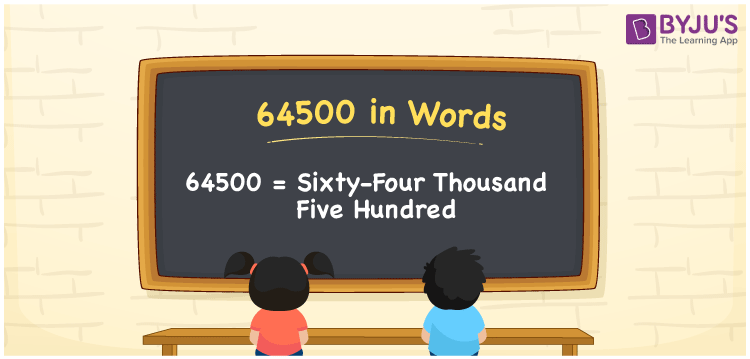# 64500 in Words

64500 in words can be written as Sixty-Four Thousand Five Hundred. If you buy school uniform material for Rs. 64500, then you can say that “I bought school uniform material for Sixty-Four Thousand Five Hundred Rupees”. You will learn in brief how to write numbers in words with the help of the English alphabet. Therefore, 64500 can be read as “Sixty-Four Thousand Five Hundred” in English.

 64500 in words Sixty-Four Thousand Five Hundred Sixty-Four Thousand Five Hundred in Numbers 64500

## 64500 in English Words## How to Write 64500 in Words?

The place value chart of the number 64500 is explained below in a systematic form to help students grasp the concepts effortlessly.

 Ten Thousands Thousands Hundreds Tens Ones 6 4 5 0 0

64500 in expanded form is:

6 x Ten Thousand + 4 x Thousand + 5 × Hundred + 0 × Ten + 0 × One

= 6 x 10000 + 4 x 1000 + 5 × 100 + 0 × 10 + 0 × 1

= 60000 + 4000 + 500

= 64500

= Sixty-Four Thousand Five Hundred

Therefore, 64500 in words is written as Sixty-Four Thousand Five Hundred.

64500 is a natural number that precedes 64501 and succeeds 64499.

64500 in words – Sixty-Four Thousand Five Hundred

Is 64500 an odd number? – No

Is 64500 an even number? – Yes

Is 64500 a perfect square number? – No

Is 64500 a perfect cube number? – No

Is 64500 a prime number? – No

Is 64500 a composite number? – Yes

## Frequently Asked Questions on 64500 in Words

Q1

### Write 64500 in words.

64500 can be written in words as “Sixty-Four Thousand Five Hundred”.
Q2

### How do you write Sixty-Four Thousand Five Hundred in numbers?

Sixty-Four Thousand Five Hundred can be written in numbers as 64500.
Q3

### Is 64500 an even number?

Yes, 64500 is an even number as it is completely divisible by 2.